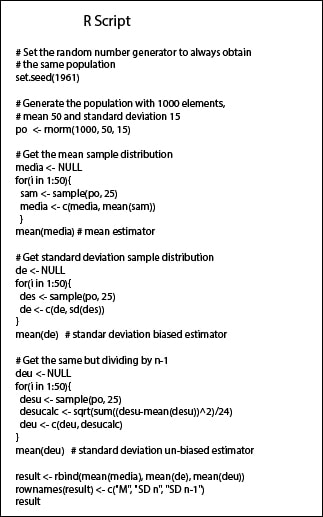# Biased and unbiased estimators.The mean one of the unbiased estimators and accurately approximates the population value. The standard deviation is a biased estimator.

Today we will talk about one of those mysteries of statistics that few know why they are what they are. I am referring to divide by n (the sample size) or by n-1 to calculate measures of and of the sample, particularly its mean (m) and its standard deviation (s).

## The estimators

The mean we all know what it is. His own name says, is the average value of a distribution of data. To calculate it we add up all the values of the distribution and divide by the total number of elements, that is, by n. Here no doubt in dividing by n to get the most utilized measure of centralization.

Meanwhile, the standard deviation is a measure of the average deviation of each value from the mean of the distribution. To obtain it we calculate the differences among each element and the mean, we square the differences to avoid that negative differences cancel out positive ones, we add them up and divide the result by n. Finally we get the square root of the result. Because it is the mean of all the differences, we have to divide the sum of all the differences by the number of elements, n, as we did with the mean, according to the known formula of the standard deviation.

However, many times we see that, to calculate the standard deviation, we divide by n-1. Why spare one element? Let’s see.We use to deal with samples, of which we get their centralization and dispersion measures. But what we are really interested in is in knowing the value of the parameters in the population from we drawn the sample. Unfortunately, we cannot calculate these parameters directly, but we can estimate them from the sample’s statistics. So, we want to know whether the sample mean, m, is a good estimator of the population mean, μ. We also want to know if the standard deviation of the sample, s, is a good estimate of the deviation of the population, which we call σ.

## An experiment

Let’s do an experiment to see if m and s are good estimators of μ and σ. We are going to use the program R. I leave the list of commands (script) in the accompanying figure in case you want to reproduce it with me.

First we generate a population of 1,000 individuals with a normal distribution with mean 50 and standard deviation of 15 (μ = 50 and σ = 15). Once done, let’s see first what happens in the case of the mean.

If we draw a sample of 25 elements from the population and calculate its mean, this mean will resemble that of the population (if the sample is representative of the population), but there will be differences due to chance. To overcome these differences, we obtain 50 different samples, with their 50 different means. These means follow a normal distribution (the so-called sampling distribution), whose mean is the mean of all that we got from the samples. If we extract 50 samples with R and find the mean of their means, we see that this is equal to 49.6, which is almost equal to 50. So we see that we can estimate the mean of the distribution using the means of the samples.

And what about the standard deviation? If we do the same (extract 50 samples, calculate their s and finally, calculate the mean of the 50 s) we get an average of 14.8 s. This s is quite close to the value 15 of the population, but it fits worse than the value of the mean. Why?

## Biased and unbiased estimators

The answer is that the sample mean is what is called an unbiased estimator of the population mean, and the mean value of the sampling distribution is a good estimate of the population parameter. However, with standard deviation the same thing does not happen because it is a biased estimator. This is because the variation in the data (which is ultimately what measures the standard deviation) is higher in the population than in the sample because the population has a larger size (larger size, greater possibility of variation). So, we divide by n-1, so that the result is a little higher.

If we run the experiment with R dividing by n-1, we obtain an unbiased standard deviation of 15.1, something closer than that obtained dividing by n. This estimator (dividing by n-1) would be an unbiased estimator of the population standard deviation. So what we use? If we want to know the standard deviation of the sample we can divide by n, but if we want to estimate the theoretical value of the deviation in the population, the estimate will fit better if we divide by n-1.

## We’re leaving…

And here we end this nonsense. We could talk about how we can get not only the estimate from the sample distribution, but also its confidence interval which includes the population parameter with a given confidence level. But that is another story…

This site uses Akismet to reduce spam. Learn how your comment data is processed.

Esta web utiliza cookies propias y de terceros para su correcto funcionamiento y para fines analíticos. Al hacer clic en el botón Aceptar, aceptas el uso de estas tecnologías y el procesamiento de tus datos para estos propósitos. Antes de aceptar puedes ver Configurar cookies para realizar un consentimiento selectivo.    Más información